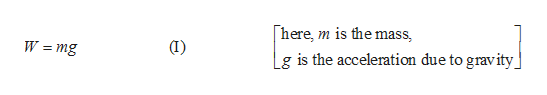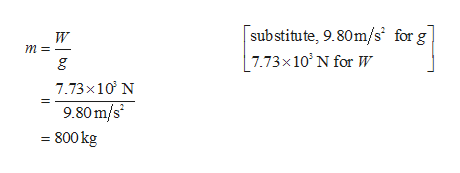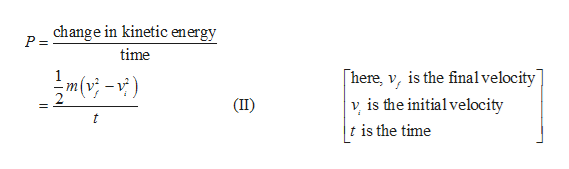# A car accelerates uniformly from rest to 24.2 m/s in 8.46 s along a level stretch of road. Ignoring friction, determine the average power required to accelerate the car if (a) the weight of the car is 7.73 x 103 N, and (b) the weight of the car is 1.90 x 104 N.

Question
49 views

A car accelerates uniformly from rest to 24.2 m/s in 8.46 s along a level stretch of road. Ignoring friction, determine the average power required to accelerate the car if (a) the weight of the car is 7.73 x 103 N, and (b) the weight of the car is 1.90 x 104 N.

check_circle

Step 1

The initial velocity of car = 0 m/s.

The final velocity = 24.2 m/s.

The time taken  by the car to accelerate =8.46 s.

(a)The power is defined as the change in energy per unit time.

According to work energy theorem, the work done is equal to the change in kinetic energy.

Let mg be the weight of the car,help_outlineImage Transcriptionclosehere, m is the mass (I) W =mg - Lg is the acceleration due to gravity fullscreen
Step 2

Rearrange equation (I) to obtain an expression for mass, and substitute the corresponding values.help_outlineImage Transcriptionclosesub stitute, 9.80m/s for g 7.73x 10 N for W W m 7.73x10 N 9.80 m/s 800 kg bo fullscreen
Step 3

Write the expressio...help_outlineImage Transcriptionclosechange in kinetic energy P = time here, v, is the finalvelocity] 1 v is the initial velocity t is the time (II) t A. fullscreen

### Want to see the full answer?

See Solution

#### Want to see this answer and more?

Solutions are written by subject experts who are available 24/7. Questions are typically answered within 1 hour.*

See Solution
*Response times may vary by subject and question.
Tagged in

### Physics